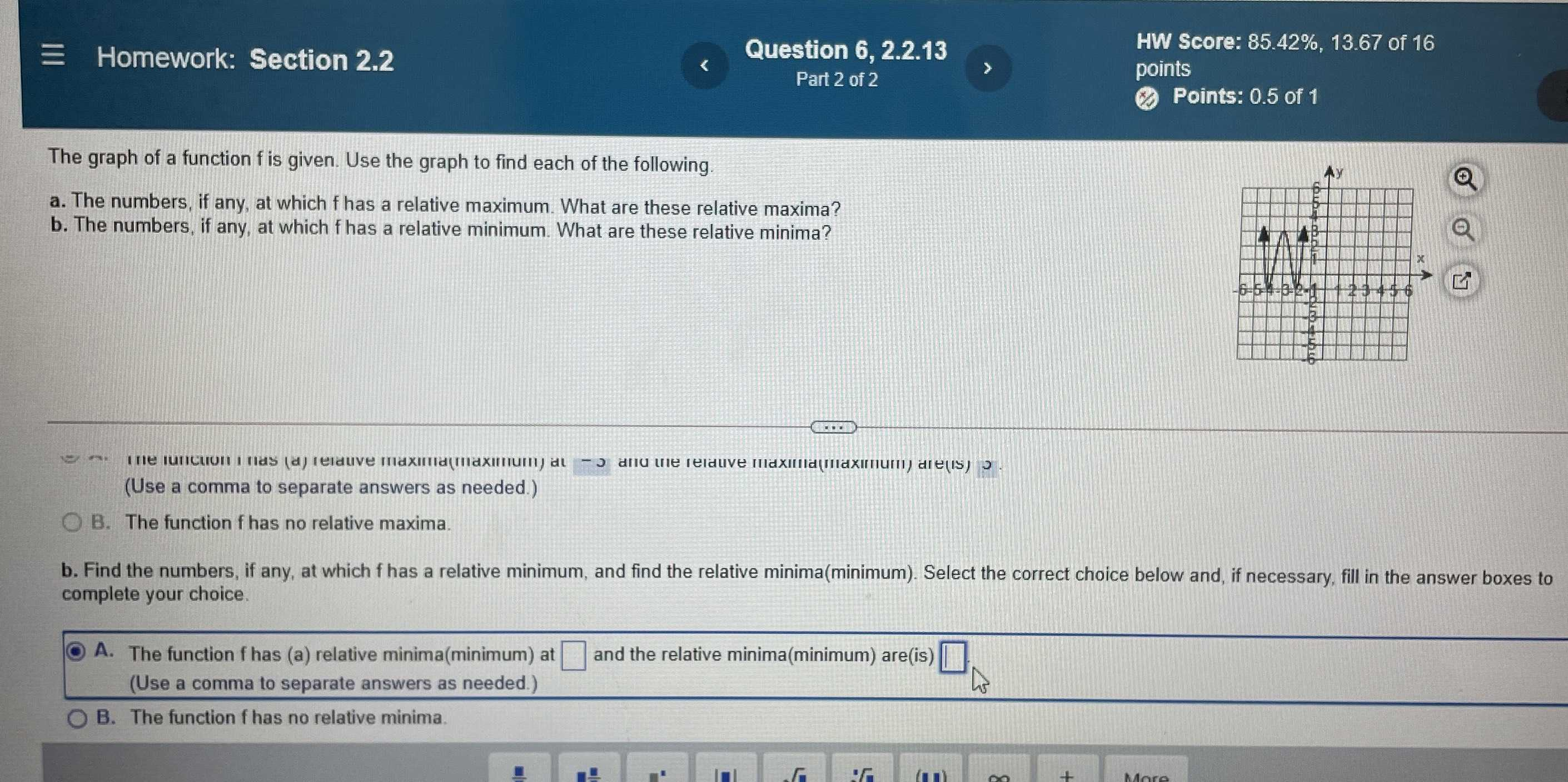### ¿Todavía tienes preguntas de matemáticas?

Pregunte a nuestros tutores expertos
Algebra
PreguntaThe graph of a function $$f$$ is given. Use the graph to find each of the following. a. The numbers, if any, at which $$f$$ has a relative maximum. What are these relative maxima? b. The numbers, if any, at which $$f$$ has a relative minimum. What are these relative minima?

A. at $$x= - 4,x= - 2$$, is $$- 1$$